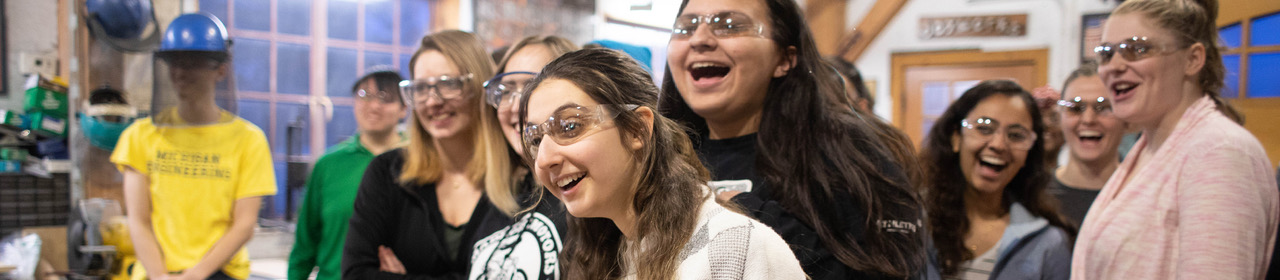•## MSE330 : Thermodynamics of Materials

##### The laws of thermodynamics and their consequences. Mass and energy balances. Gas reactions. Equilibrium. Phase diagrams. Ellingham, Pourbaix and stability diagrams.

Prerequisites: (CHEM 130 or 210) and (PHYSICS 140 and 141) and MATH 215 and (MSE 220 or 250)
Instructor: Ashwin Shahani

### Course Topics:

1. The First Law of Thermodynamics
2. The Second Law of Thermodynamics
3. Statistical interpretation of entropy
4. Enthalpy and the Helmholtz and Gibbs energies
5. Heat capacity, enthalpy, entropy, and the Third Law of Thermodynamics
6. Phase equilibrium in a one-component system
7. The behavior of gases
8. The behavior of solutions
9. Gibbs energy vs. composition and phase diagrams of binary systems
10. Reactions involving gases
11. Reactions involving pure condensed phases and a gaseous phase
12. Reaction equilibria in systems containing components in condensed solution

### Course Objectives:

1. To teach students the nature and basis of the laws of thermodynamics.
2. To teach students when and how to apply the laws of thermodynamics to materials, how to calculate the changes in the thermodynamic variables when a material changes state (from gas to liquid to solid) or when the temperature of a material and/or the pressure acting on the material is changed without a change in state.
3. To teach students the thermodynamics of mixtures and phases, the coexistence criteria for distinct phases, and how to use phase diagrams to succinctly summarize mixing behavior.
4. To teach students the thermodynamics of the reactions of solids with gases and the reactions of several materials all in condensed phases.

### Course Outcomes:

1. Given heat capacity data for reactants and products and the heat of reaction at one temperature, compute the heat of reaction at any other temperature [1,2].
2. Given that a gas can be assumed to be approximately ideal, be able to determine work and heat in and out of the gas as it is cycled over a range of temperatures and pressures [1,2].
3. Given component activities in a solution at one temperature and concentration, compute the activities at another temperature and concentration assuming the solution is regular .
4. Given ideal solution behavior, be able to compute changes in enthalpy and Gibbs energy when a pure material is added to the solution [1,3].
5. Given vapor pressure data of a component, be able to compute boiling points and latent heat of boiling .
6. Given the activity coefficient of one component in a binary mixture over the range of composition, be able to determine the activity of the second component at any composition .
7. Given appropriate thermodynamic data, be able to determine composition in a gas mixture at any specified temperature, and be able to compute equilibrium compositions when in contact with solids with similar components .

### Assessment Tools:

1. In-class reflections and closed-book quizzes and exams to test objectives for individual students.
2. Weekly problem sets to test objectives with less time pressure and with the possibility of student collaboration for conceptualization of solution.